From 2D to 3D Bifurcation Structures in Field Oriented Control of a PMSM | OMICS International
Journal of Electrical & Electronic Systems

# From 2D to 3D Bifurcation Structures in Field Oriented Control of a PMSM

Wahid Souhail1*, Hedi Khamari2 and Mohamed Faouzi Mimouni1

1Electrical Engineering Department, National Engineering School of Monastir Monestir, Ibn al Jazar, Tunisia

2Computer Department, Faculty of Computer Science, Taief University Taief, Saudi Arabi

*Corresponding Author:
Wahid Souhail
Electrical Engineering Department
National Engineering School of Monastir Monestir
Ibn al Jazar 5019, Tunisia
Tel: 73500515
E-mail: [email protected]

Received Date: August 30, 2016; Accepted Date: September 09, 2016; Published Date: September 16, 2016

Citation: Souhail W, Khamari H, Mimouni MF (2016) From 2D to 3D Bifurcation Structures in Field Oriented Control of a PMSM. J Electr Electron Syst 5: 196. doi: 10.4172/2332-0796.1000196

Copyright: © 2016 Souhail W, et al. This is an open-access article distributed under the terms of the Creative Commons Attribution License, which permits unrestricted use, distribution, and reproduction in any medium, provided the original author and source are credited.

Visit for more related articles at Journal of Electrical & Electronic Systems

#### Abstract

This paper presents new aspects of bifurcation structures in a Permanent Magnet Synchronous Machine (PMSM) in both motor and generator operating modes.
It considers the case where the PMSM speed is regulated with a Field-oriented control (FOC). A period doubling bifurcation cascade under the variation of a proportional control parameter leading to chaotic states, was identified.
The investigation of parametric singularities allows us to identify a complex bifurcation structure including three generic bifurcations. Such structure is made of the Limit Point (LP), the Hopf (H) and the Bogdanov-Takens (BT) bifurcation sets. Sufficient conditions of the existence of the main bifurcations are given analytically.
An overview of the phase space singularities associated to the parametric singularities is presented. Moreover, embedding 2D bifurcation sets in a 3D parametric space, led to identify certain bifurcation surface structures. The bifurcation surfaces established correspond to limit point bifurcation for the motor and generator operating mode, and for period doubling period bifurcation. The 3D bifurcation sets play an important role to study the combined effect of three different parameters on the PMSM dynamics, and permit to control the complex PMSM dynamics to a stable equilibrium dot by an adequate tuning of either control and system parameters.

#### Keywords

Bifurcation surface; Limit point; Hopf; Bogdanov-Takens; PMSM dynamics

#### Abbreviations

Ω : mechanical angular speed (rad/s); id,q: direct and quadrature-axis currents (A); vd,q : direct and quadrature axis voltages (V); TL: load torque (N.m); Ld,q: direct and quadrature-axis inductance (H); Rs: stator winding resistance (ohm); φφ: permanent magnet flux (wb); f : viscous damping coefficient (N/rad/s); J: polar moment of inertia (kgm2); p: number of pole pairs of the rotor; kp: proportional constant of current regulator; ki : integral constant of current regulator; kpw : proportional constant of speed regulator; ref Ù : reference speed input; idref: reference current input.

#### Introduction

The permanent magnet synchronous motors (PMSM) are among the main preferences for industrial control applications. It has high power density, fast dynamic response and high efficiency. Because of its high reliability, the Field -oriented based controller (FOC) is mainly used for high dynamic performance induction motor drives.

For several industrial implementations, the speed regulation of the PMSM is ensured with such type of controllers .

Sustained oscillations may arise near equilibrium in PMSM due to the nonlinearities inherent to it. Many undesirable behaviors of PMSM were well documented, but little understood.

According to many studies, detection and suppression of undesirable behaviors namely chaos and sustained oscillations in PMSM are of high interest [2-6].

Power systems including electromechanical machines present several challenging problems related to the nonlinear characteristics of its components. The resulting physical behaviors, including bifurcation, chaos, resonance, voltage collapse, may cause the loss of stability and the transition from normal to anomalous operating regimes [7-9]. Bifurcation theory not only renders some machines’ defaults more comprehensible but uncovers new problems that need research.

In attempts to study the dynamics of electric machines, the phase space singularities as well as the parametric singularities were studied in order to define the stability domains and to avoid the undesirable behaviors [2,4,5,10-13].

To overcome control draw backs and to solve the stabilization control problems in PMSM some control techniques were reported to be efficient enough to bring order to PMSM. The Lyapunov exponent based controller and the neural and back stepping techniques based nonlinear controllers were developed mainly to suppress chaos and to force the machine to a desired solution in an attraction basin [2-4,14-16]. In many nonlinear systems, Chaos can be originated from a succession of doubling period (Flip) bifurcations [17,18], these complex behaviors are usually twin. Thus not only chaos, but bifurcation control techniques are required for maintaining a system’s behavior in a nominal operating state and to avoid loss of stability.

On the basis of parametric plane bifurcation structures, a qualitative characterization of bifurcation structure in 3D parametric space will be developed. This allows one to explore the eventual existence of generic characteristic bifurcation structures of PMSM behavior.

Among the objectives of this study, is to find bifurcation structures in 3D-parametric space which, on one hand give a global vision on the parameter effects on PMSM behavior, and, on the other hand, define normal operating domains of PMSM. For that purpose, one can start by detecting a bifurcations in a 2D-parameteric plane, and then embed it in a 3D-parameteric space by varying a third parameter. The resulting structure is a bifurcation surface.

The numerical continuation methods are the main tool used to plot the bifurcation diagrams and to explore the dynamics of the PMSM submitted to a FOC.

Section 2 is devoted to describe the system model and to define analytically the corresponding control model. Successive period doubling bifurcation leading to chaotic behavior is presented in section 3.

The section 4 is reserved to describe the existence conditions of three generic bifurcations, namely limit point (LP), Hopf (H) and Bogdanov-Takens (BT) bifurcations for particular sets of system and control parameters.

A different approach to characterize the PMSM dynamics, based on embedding a 2D-bifurcation structure into a 3D-parametric space is presented in section 5, and then the paper is ended by some concluding remarks.

#### Mathematical Model of PMSM Drive System and Preliminaries

Consider a PMSM submitted to a Field Oriented Control (Figure 1).

The currents ia, ib are measured with a current sensor. The Clarke transform is applied to determine the projection of stator current in a two coordinate non-rotating frame. Then its projection in the (d, q) rotating frame is performed via Park transform. The d, q projection of the stator phase currents are then compared to their reference values iqref and idref = 0 and corrected by mean of PI current controllers. The outputs of the current controllers is transformed by an inverse Park transformation back from the d-q reference frame into the 2-phase system fixed with the stator which in turn is applied to the motor using the space vector modulation technique. Control of the motor speed is ensured by a reference current iqref generated by an outer loop.

The mathematical model of PMSM is given by: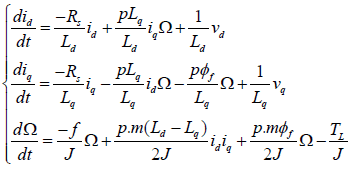(1)

For investigation of the control problem of the PMSM with smooth air gap, the direct and the quadratic-axis winding inductances are equal (L=Ld=Lq)

The PI regulator is defined by the expression: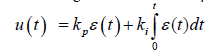(2)

Where,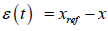(3)

The classical control method includes three PI regulators to generate the voltages vd, vq having the following expressions: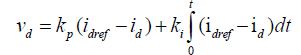(4)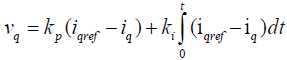(5)

The reference current iqref generated by the PI regulator of angular speed is given as: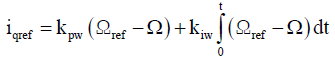(6)

The integral regulators of the system are defined as: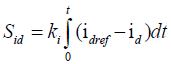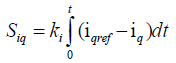(7)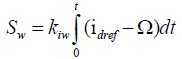The expression (6) becomes: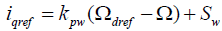(8)

The input voltages are expressed as: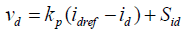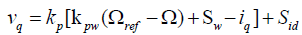(9)

Differentiating the equations (7) with respect to t, gives the following differential system: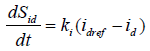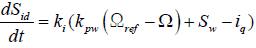(10)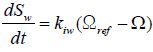Then, the machine control model is described by a system of six differential equations: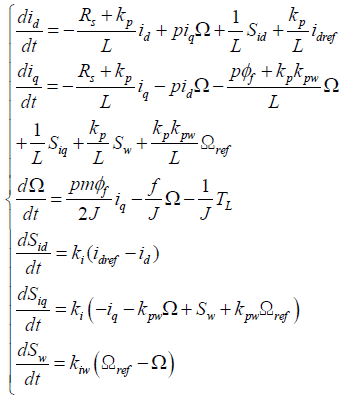(11)

#### Periodic Doubling Bifurcation and ‘Route’ to Chaos

Computation of equilibrium points

The equation (11) can be written in the form: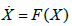(12)

With X=(x1,x2,x3,x4,x5,x6)=(id,iq, Ω, Sid, Siq, Sω) and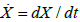The equilibrium points are obtained by equating the righthand side of the equation (12) to zero as follows: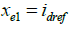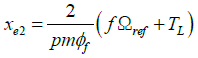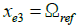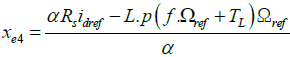(13)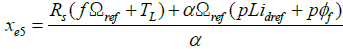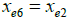with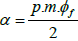The global stability and dynamic characteristics of the equilibrium point are profoundly affected by the parametric singularities namely the bifurcation phenomena that will be discussed in the next sections.

Period doubling bifurcation of limit cycles

Analysis of the dynamical behavior of the PMSM led to identify a sequence of particular set of control bifurcation points. The transition from an equilibrium point to a limit cycle through a Hopf bifurcation is followed by a cascade of doubling period bifurcations (PD) which constitutes a veritable route to chaos.

A doubling period bifurcation occurs when a branch of perioddoubled solutions is created or destroyed at the critical point. In the first case if the Hopf bifurcation is supercritical, a branch of stable perioddoubled solutions emerges and the original stable periodic solutions will be continued as a branch of unstable periodic solutions. In the second case, if the Hopf bifurcation is subcritical, an unstable perioddoubled solution is destroyed and then the stable periodic solutions evolve on a branch of unstable periodic solutions .

For the following conditions:

• input reference ωref= 100rad s−-1, and idref = 0A

• The control parametres are kpw=0.001, kp=0.25, ki=1, kiw=20

The machine has an equilibrium point xe = (0.015, 6.185,1 00, 1.682, 6.306, 6.245) with the eigenvalues : λ1,2 = −0.0539 ± j0.0528, λ3,4 = −0.0106 ± j0.0097, λ5 = −0.0026, λ6=-0.003.

The continuation of this equilibrium point by varying kp led to a supercritical Hopf bifurcation at = 0.596 kp with the eigenvalues

λ1,2 = −0.00757 ± j0.00428, λ3 = −0.000899

λ4 = −0.000287, λ5,6 = ± j0.00428.

Thus, the resulting limit cycle, with period T=9.33 s is stable and will undergo a cascade of period doubling bifurcation by decreasing kp Figure 2. As the parameter kp is varied, the machine enters into a complicated dynamics, through period doubling bifurcation, chaos intermittency and so on. Based on simulation results, the coordinates and the eigenvalues of the different PD bifurcation points are given in Table 1. It appears that in the PD critical points, two eigenvalues are equal to -1.

Figure 2: Bifurcation diagram of x1 versus kpna : Sequence of period doubling and route to chaos.

Bif point kp Initial conditions Eigenvalues
PD1 4.59196 (-6.824, 2.423711,-1.84422, 0.0034, -0.0015, 1.004)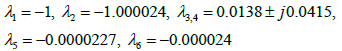PD2 4.55175 (-6.4432, 2.53505,-1.7974,0.003620,-0.0013, 1.0048)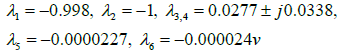PD3 4.55175 (-7.2113, 2.3206,-1.9085, 0.003235,-0.0016, 1.0036)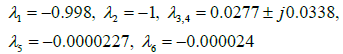PD4 4.539713 (-6.268,2.47,-1.78,0.003651,-0.0012, 1.0049)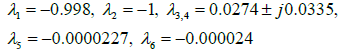PD5 4.539713 (-6.64948, 2.5556,-1.8128, 0.003625,-0.0013,1.0048)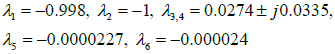PD6 4.539713 X=(-7.21649, 2.3515,-1.9036,0.003331,-0.0015, 1.0038)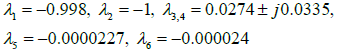PD7 4.539713 X=(-7.27319,2.2835,-1.9234,0.003149,-0.0016, 1.0033)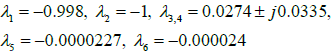Table 1: Coordinates of the PD bifurcations points.

To make an overall inspection of the machine dynamics in different points of the bifurcation diagram, namely A, B, C, D, E, F, G, H and I, the phase portraits of the coordinate x1 versus the variable x2 are plotted in Table 2.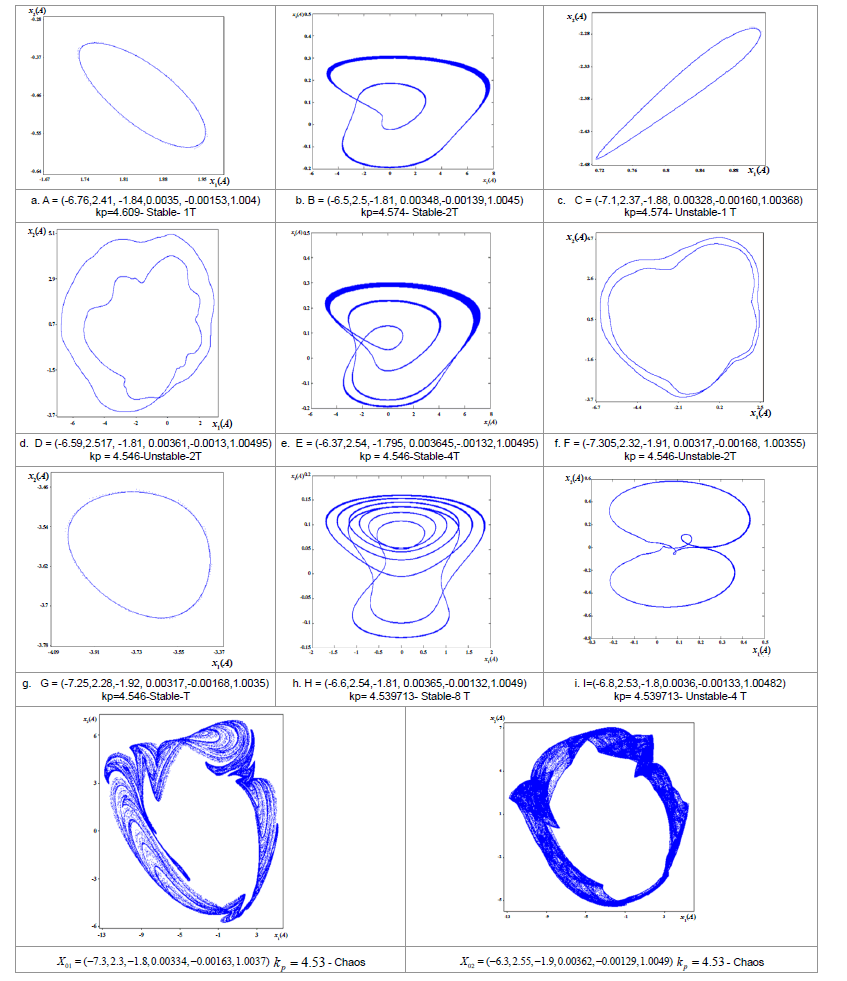Table 2: Phase portraits in phase plane x1−x2 for different values of kp.

#### Existence Conditions of Certain Parametric Singularities

Some control approaches reset the Integral action of the PI when the Saturation is reached and particularly the anti wind up methods based on removing the integral part from the input .

The integral correctors of the machine drive have an important effect on the system dynamics. The case of a machine driver without integral correctors is considered, so the mathematical model of system given in (11) can be transformed as: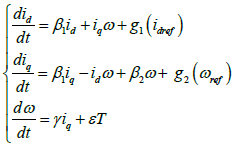(14)

With: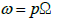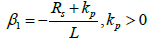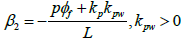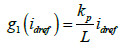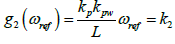With: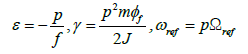For graphs, let k2 parameter denote the function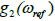The Jacobian matrix of the system (14) is defined as: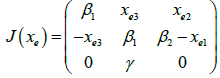(15)

The characteristic polynomial of Jacobian matrix is: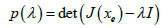(16)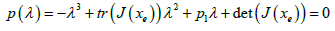(17)

Where,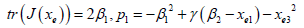and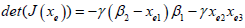Preliminary results on existence conditions of three bifurcation types sets for certain sets of PMSM and control parameters are determined in the following subsections.

Limit point bifurcation

Investigation was conducted to establish the conditions leading to limit point bifurcation. The LP bifurcation, which results from interaction between stable and unstable equilibrium points, has three eigenvalues, one of which is 0 and two are nonzero. Therefore, the necessary existence condition is derived from the equation p (λ)=0, by taking det (J(xe))=0, therefore the roots of the characteristic polynomial are expressed as: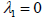and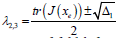sucha as,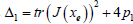Now, the detection of fold bifurcation point needs to search the roots of the determinant equation.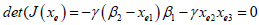(18)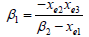With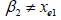Hopf bifurcation

A Hopf bifurcation occurs when two complex eigenvalues cross through the imaginary axis while the other eigenvalues are in the open left-hand side of the complex plane.

The Routh-Hurwitz stability criterion is applied to polynomial p(λ) , in order to derive the condition for existence of Hopf bifurcation  (Table 3).

 λ3 -1 p1 λ2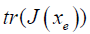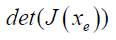λ1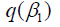0 λ0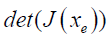0

Table 3: Routh-Hurwitz criterion.

With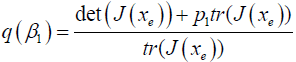(19)

Such condition is stated as: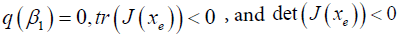Then,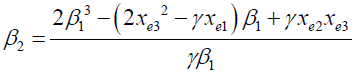(20)

Where,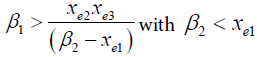Bogdanov-Takens bifurcation

The Bogdanov-Takens bifurcation (BT) is a local codimension 2 bifurcation of an equilibrium point. In the parameter plane, the critical equilibrium has a zero eigenvalue of multiplicity two. For near parameter values, the system has a saddle and a non-saddle points which collide and disappear via a saddle node bifurcation. The non-saddle equilibrium turns into a limit cycle when it crosses an Andronov-Hopf bifurcation H. This cycle changes into an orbit homoclinic to the saddle and vanishes via a saddle homoclinic bifurcation .

Looking for the possible existence of double-zero eigenvalues of the Jacobian matrix (18), the necessary condition for the occurrence of such a bifurcation is: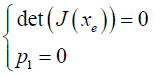Then the characteristic polynomial becomes: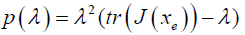(21)

The eigenvalues are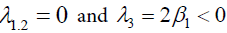The existence of Bagdanov-Takens bifurcation BT is controlled by the condition: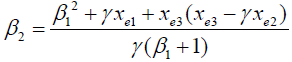Thus,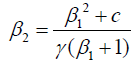(22)

With,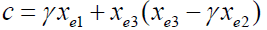#### Bifurcation Structure Analysis

Using bifurcation calculations one can divide a two-parameter plane into a number of regions, for which there are qualitatively different dynamics. This paves the way to classify and further investigate the dynamical behavior in each of these regions.

Machine operation under overload mode (TL>TLn)

For a load torque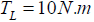and starting from the initial condition xi =(1,1,1) , the trajectories of state variables converge to the equilibrium point: xe =(-2.45,8.1,73.76) where the inputs of the system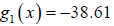and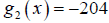. Fixing the parameter of system at β1 = −5 and β2 = −10 , the eigenvalues of this equilibrium point are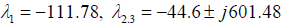. (Figure 3) displays the parameter plane in which the system behavior is investigated in different areas. The Bogdanov-Takens bifurcation point is detected for β1 = −10 and β2 = −3.83. The corresponding eigenvalues are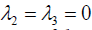and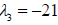. The area 2 presents an unstable dynamical behavior of the system where three eigenvalues are positives.

The limit point curve Lp+ is controlled by λ2=0,λ1 is negative and λ3 is in the right half of the complex plane. The corresponding behavior is anunstable equilibrium point which appears also in areas 2’ and 2”. The areas 2 and 2’ are separated by a limit point curve Lp+.

The areas 1’ and 1 which are characterized by a stable equilibrium point, are delimited by a Limit Point curve Lp (the eigenvalues λ12 are negatives and λ3 = 0 ), a Hopf bifurcation curve H (λ2 , λ3 are purely imaginary and λ1 is negative) and a zero neutral saddle curve NS. Each point of such bifurcation curve has one eigenvalue equal zero λ1 = 0 and two opposite eigenvalues λ2 + λ3 = 0 .

Machine Operation for (TL≤TLn)

Now, if the system parameters are changed to β1= −100, β2 = −46.66, γ = 8100 and starting from the same initial point xi=(1,1,1), the machine will tend to the equilibrium point EP : x = (−0.99, 0, 2.18) and the inputs of the motor will be g1(x)=-100, g2(x)=100 . TL=2N.m and the eigenvalues of the Jacobian matrix are λ1 = −339.33 and λ2,3 = −170, 22 ± j584.29 .

One parameter bifurcation diagram: Varying the parameter β1 , limit point bifurcations are detected and displayed in(β1,x1 ) plane (Figure 4).

The type of bifurcation point is detected at β1 = −10.1 with the eigenvalues λ1,2 = −10.5 ± j129.02 and λ3 = 0 and an equilibrium point x = (−2.42, − 0.019, 73.76) .

In (Figure 5), taking the load torque TL as a bifurcation parameter, four bifurcations of type LP are detected in (TL,x1) plane (Table 4).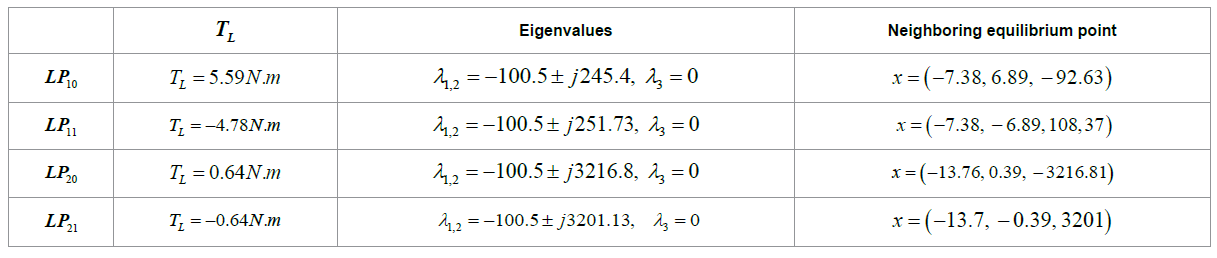Table 4: Limit points: These points can be used as starting points for tracing bifurcation curves in any parameter plane including TL.

Two-parameter bifurcation plane: The continuation of the limit points LP10 and LP11 in the parameter plane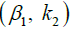permits to trace limit point bifurcation curves for motor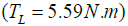and generator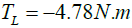operating modes, (Figure 6).

Both curves contain a branching point (BP) and a Bogdanov- Takens bifurcation points.

For the motor operating mode the branch point is: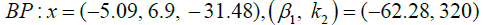and the eigenvalues are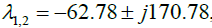and λ3=0. In this singular point two equilibrium points collide and disappear and there is no unique tangent, which results in speed control lost. The Bogdanov-Takensfor the same operating mode is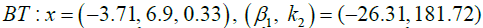and the eigenvalues are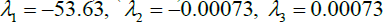. In this point the motor tends to reverse rotation and continues to spin at low speed x3 = 0.33rad/s

For generator operating mode, the same types of bifurcations are shown in an apparent symmetry on the LP bifurcation curve.

Effect of the variation of a third parameter on the bifurcation sets: More features of the bifurcation structure displayed in a parameter plane could be revealed by varying a third parameter. In the parameter plane (kp,k2), a set of LP bifurcation curves are computed for different values of kp and for both operating modes (Figure 7). The coordinates of branch points and Bogdanov-Takens bifurcation for a motor operating mode are given in (Tables 5 and 6).

 kp 5 10 15 20 25 30 35 40 k2 271.87 320.65 345.42 360.42 370.48 377.69 383.115 387.34 β1 -51.92 -62.32 -67.62 -70.83 -72.99 -74.54 -75.54 -76.61

Table 5: Branch point bifurcation coordinates.

 kp 5 10 15 20 25 30 35 40 k2 165.52 181 189.28 193.66 196.52 198.54 200.103 201.19 β -23.97 -26.31 -27.41 -28.048 -28.46 -28.75 -28.97 -29.14

Table 6: Bogdanov-Takens bifurcation coordinates.

Embedding the bifurcation structure shown in Figure 8 into the parametric space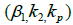(Figure 8a). A qualitative 3D representation of the LP bifurcation curves for each of the motor operating mode (blue) and the generator operating mode (green), shows a parabolic shaped surface in the parametric space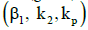.

Each bifurcation surface includes, for each operating mode, a Branch Point bifurcation curve (red dashed line) and a Bogdanov- Takens bifurcation curve (black dashed line). The continuity of the transitions between the LP bifurcation curves is let to be proved in further researches. The LP bifurcation curves under the variation of kp form a 3D parabolic shaped surface concave to the left for motor operating mode and concave to the right for generator operating mode (Figure 8b).

A similar analysis is applied to determine a period doubling bifurcation surface. Starting from a set of closed PD bifurcation curves for different values of kiw, traced in the parametric space (kp, ki, kiw), a qualitative 3D closed PD bifurcation surface is derived, see Figures 10 and 11 respectively. The construction of bifurcation surface is introduced, in order to put forward a new formalism related to bifurcation structure in 3D parametric space. As a bifurcation point of codimension 1 map to a bifurcation curve in parameter plane, the latter maps to a surface in 3D parametric space. A codimension two bifurcation point in parameter plane, maps to a bifurcation curve in 3D parameter space. Based on the results obtained in Figure 8 and under realistic assumptions, the 3D parameter space can be considered as a set of codimension one bifurcation surfaces connected through codimension two bifurcation curves. This study paves the way to further researches aiming to explore more generic structures of bifurcation surfaces in 3D parametric space and to study the effect of varying a fourth parameter on their shapes and sizes. Also the study aims to strengthening the knowledge and practice on the combined effect of a broader set of parameters on the PMSM dynamics (Figures 9 and 10).

#### Conclusion

The simulation results not only can reveal the dependence of PMSM behavior on the control parameters, but also it can be used for control and design purposes. Methods from bifurcation theory are applied to identify and characterize complex bifurcation sets of PMSM behavior in both motor and generator operating modes.

After identifying a period doubling bifurcation cascade under the variation of a proportional control parameter, the analytical necessary conditions for existence of Hopf and Bogdanov-Takens and limit point bifurcations are given in this paper. These parametric space singularities permit to understand the mechanism of transition from equilibrium dot to limit cycle and from one limit cycle to another limit cycle with different order and stability.

Embedding 2D bifurcation structures into 3D bifurcation ones is mainly introduced to study the combined effect of a larger set of parameters on the PMSM dynamics and to widen the understanding of certain types of parametric and phase space singularities.

The bifurcation surfaces established correspond to limit point bifurcation for the motor and generator operating mode, and for period doubling period bifurcation.

#### References

Select your language of interest to view the total content in your interested language

### Article Usage

• Total views: 9592
• [From(publication date):
September-2016 - Jan 19, 2020]
• Breakdown by view type
• HTML page views : 9439Can't read the image? click here to refresh